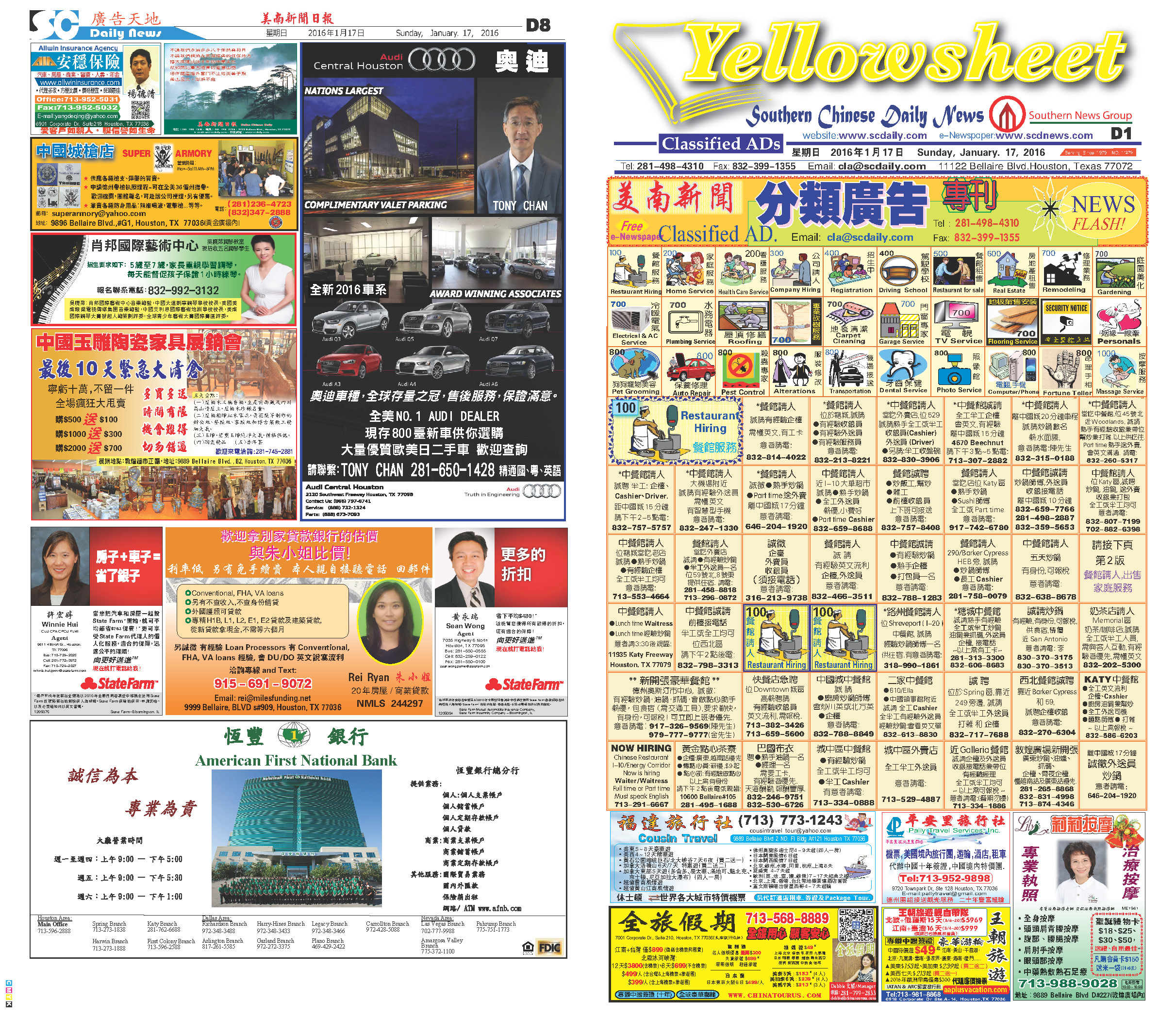160123 Epaper

 A Section B SectionC Section D Section160122 Epaper

 A Section B SectionC Section D Section160121 Epaper

 A Section B SectionC Section D Section160120 Epaper

 A Section B SectionC Section D Section160119 Epaper

 A Section B SectionC Section D Section160118 Epaper

 A Section B SectionC Section D Section160117 Epaper

 A Section B SectionC Section D Section160116 Epaper

 A Section B SectionC Section D Section160114 Epaper

 A Section B SectionC Section D Section160115 Epaper

 A Section B SectionC Section D Section160113 Epaper

 A Section B SectionC Section D Section